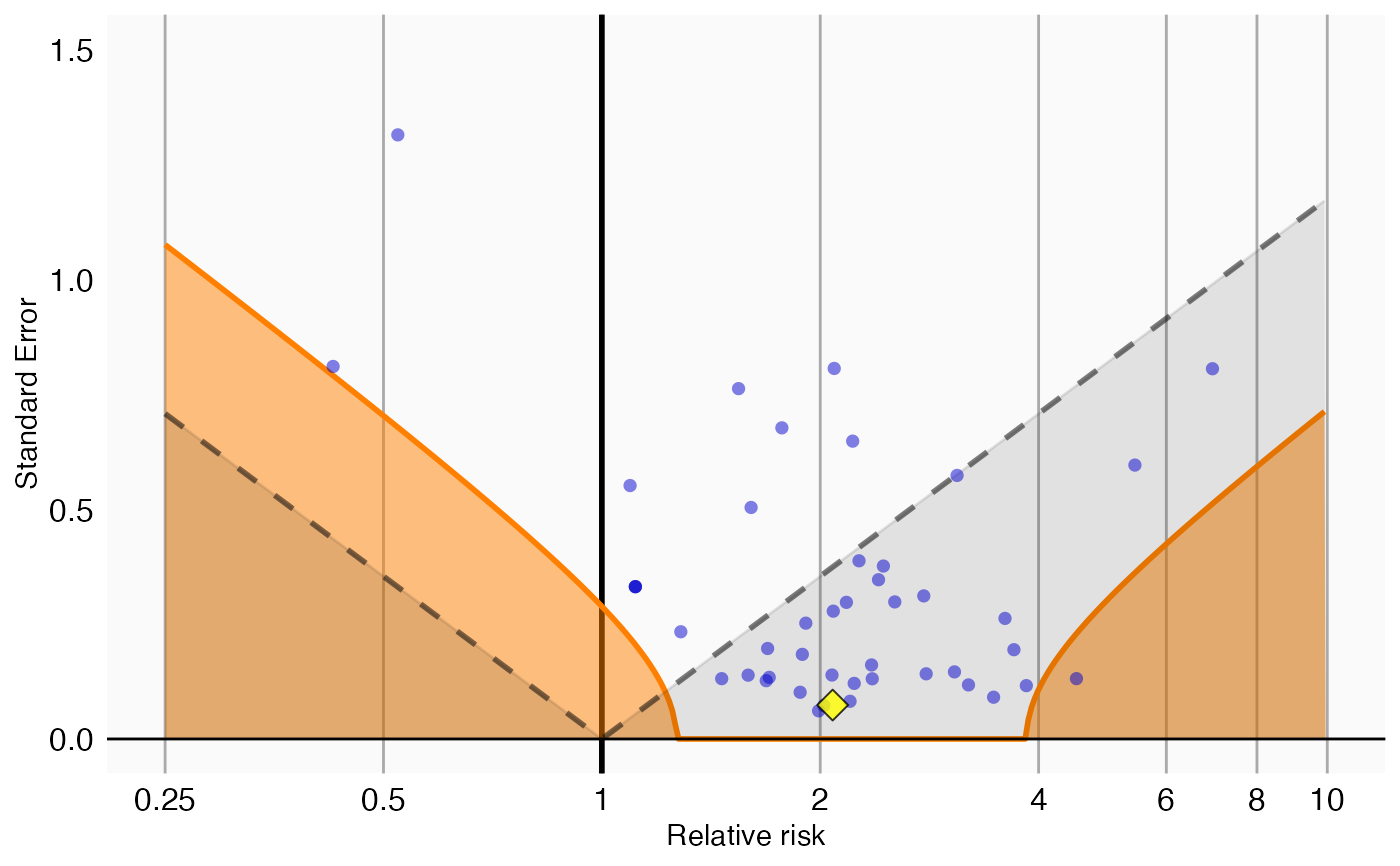plotCalibrationEffect creates a plot showing the effect of the calibration.

plotCalibrationEffect(
logRrNegatives,
seLogRrNegatives,
logRrPositives = NULL,
seLogRrPositives = NULL,
null = NULL,
alpha = 0.05,
xLabel = "Relative risk",
title,
showCis = FALSE,
fileName = NULL
)

## Arguments

logRrNegatives A numeric vector of effect estimates of the negative controls on the log scale. The standard error of the log of the effect estimates of the negative controls. Optional: A numeric vector of effect estimates of the positive controls on the log scale. Optional: The standard error of the log of the effect estimates of the positive controls. An object representing the fitted null distribution as created by the fitNull or fitMcmcNull functions. If not provided, a null will be fitted before plotting. The alpha for the hypothesis test. The label on the x-axis: the name of the effect estimate. Optional: the main title for the plot Show 95 percent credible intervals for the calibrated p = alpha boundary. Name of the file where the plot should be saved, for example 'plot.png'. See the function ggsave in the ggplot2 package for supported file formats.

## Value

A Ggplot object. Use the ggsave function to save to file.

## Details

Creates a plot with the effect estimate on the x-axis and the standard error on the y-axis. Negative controls are shown as blue dots, positive controls as yellow diamonds. The area below the dashed line indicated estimates with p < 0.05. The orange area indicates estimates with calibrated p < 0.05.

## Examples

data(sccs)
negatives <- sccs[sccs$groundTruth == 0, ] positive <- sccs[sccs$groundTruth == 1, ]
plotCalibrationEffect(negatives$logRr, negatives$seLogRr, positive$logRr, positive$seLogRr)